# What is the Integral Test for Convergence?

Katherine Kaylegian-Starkey, Cameron Smith, Kathryn Boddie
• Author
Katherine Kaylegian-Starkey

Katherine has a bachelor's degree in physics, and she is pursuing a master's degree in applied physics. She currently teaches struggling STEM students at Lane Community College.

• Instructor
Cameron Smith

Cameron has a Master's Degree in education and has taught HS Math for over 25 years.

• Expert Contributor
Kathryn Boddie

Kathryn has taught high school or university mathematics for over 10 years. She has a Ph.D. in Applied Mathematics from the University of Wisconsin-Milwaukee, an M.S. in Mathematics from Florida State University, and a B.S. in Mathematics from the University of Wisconsin-Madison.

Understand what a convergent and a divergent integral is. Learn how to use the integral test for convergence to find out if a series converges, and work through examples. Updated: 02/05/2022

Show

## Integral Convergence

Recall that one condition of the Fundamental Theorem of Calculus, is that the interval being investigated must be a closed interval. In mathematics and science, however, it is not uncommon to consider integrals that have one or both boundaries at +/- infinity. Integrals that are being considered on open intervals are called improper integrals, and to evaluate an improper integral, the limit of the integral is evaluated for convergence or divergence. Integral convergence is defined as an integral whose limit exists and is finite, and integral divergence is defined as an integral whose limit is either +/- {eq}\infty {/eq}, or nonexistent. When evaluating an integral with one boundary at infinity,

{eq}\int_a^{\infty} f(x) dx = lim_{A \rightarrow \infty} \int_a^{A} f(x) dx {/eq}.

If both of the boundaries of the integral are infinity, the integral must be broken up, such as

{eq}\int_{-\infty}^{\infty} f(x) dx = \int_{-\infty}^{a} f(x) dx + \int_{a}^{\infty} f(x) dx {/eq},

and both terms on the right must be evaluated for convergence or divergence. If either term is divergent, then so is then entire integral.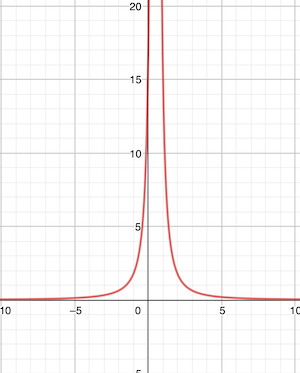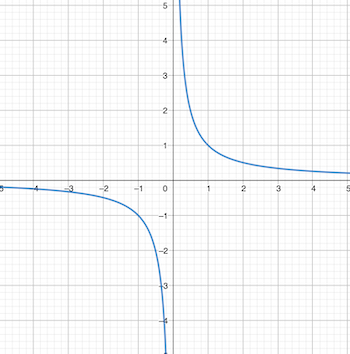Figure 1 is the graph of {eq}f(x) = \frac{1}{(0.5x-0.3)^2} {/eq}. Notice, on both sides of the peak, the function goes to 0. This is an example of a function that will have a convergent integral. Figure 2, on the other hand, is the graph of {eq}f(x) = \frac{1}{x} {/eq}. Notice on this graph that the function can be followed to +/- {eq}\infty {/eq}, without ever touching the x-axis. This is an example of a function whose integral is divergent.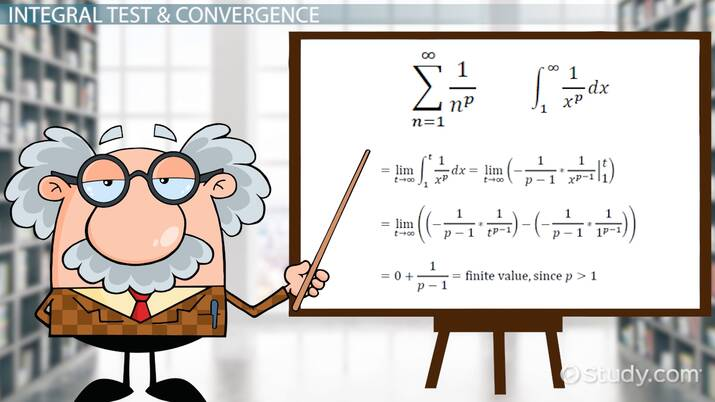An error occurred trying to load this video.

Try refreshing the page, or contact customer support.

Coming up next: Using the Ratio Test for Series Convergence

### You're on a roll. Keep up the good work!

Replay
Your next lesson will play in 10 seconds
• 0:04 The Integral Test
• 1:44 Integral Test & Convergence
• 2:49 The Integral Test and P-Series
• 3:45 Lesson Summary
Save Save

Want to watch this again later?

Timeline
Autoplay
Autoplay
Speed Speed

## Integral Test for Convergence

What is the integral test? The concepts of integral convergence and divergence are extended to the study of mathematical series, in the form of the integral test for convergence. Recall that a series is a summation, and mathematical series, written as {eq}\sum_n^i f(n) {/eq}. This summation can be rewritten as an integral, {eq}\int_n^i f(x) dx {/eq}, and the connection between series and integrals makes it possible to use integral tests on series. If a series is written as {eq}\sum_n^{\infty} f(n) {/eq}, such that the summation is taken to infinity, it is possible to tell whether or not the series converges, by using the integral test for series. To use the integral test for convergence, the integral test rules state that the series must be continuous and decreasing. If these two conditions are met, testing for convergence can be computed as follows:

1) Write the series as an integral where f(x) is the integrand, and the limits of the series are the boundaries of the integral:

{eq}\sum_n^{\infty} f(n) = \int_n^{\infty} f(x) dx {/eq}.

2) Change the limits of integration on right side of 1):

{eq}\int_n^{\infty} f(x) dx = \int_n^{N} f(x) dx {/eq}.

3) Perform the integration:

{eq}\int_n^{N} f(x) dx = F(x) |_n^N {/eq}

4) Take the limit of the result of the integration:

{eq}lim_{N \rightarrow \infty} F(N) - F(n) {/eq}

If the limit is finite and it exists, the series converges. If the limit is infinity or the limit does not exist, DNE, the series diverges.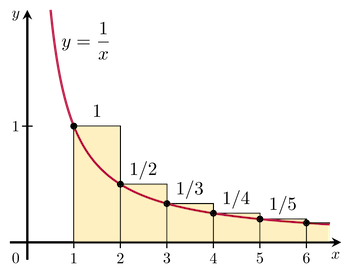### Integral Test Conditions

The integral test for convergence can be used because taking the limit of a Riemann's sum for a function f(n), is analogous to taking the integral of the function f(x). This means, that if an integral is convergent, its associated series is too, but finding the convergence of the integral and series is not the same as finding the sum of the series. If an integral, or a series, is bounded and monotonically decreasing, or decreasing such that each successive value is less than or equal to the one before, it is always convergent. If these conditions are not met, then to find out whether or not the series and its associated integral are convergent or not, the integral test conditions must be met:

1) the series f(n) must be continuous on the interval {eq}[ n, \infty) {/eq}

2) the series f(n) must be monotonically decreasing

3) the starting value of the series must be the lower boundary of the integral

### Integral Test Examples

The following integral test examples show how to prove whether or not certain series are convergent or divergent.

Example 1: Prove that the harmonic series {eq}\sum_{n=1}^{\infty}\frac{1}{n} {/eq} is divergent.

1) Rewrite the series as an integral.

{eq}\sum_{n=1}^{\infty}\frac{1}{n} = \int_1^{\infty} \frac{1}{x} dx {/eq}

2) Adjust the boundaries of the integral.

{eq}\int_1^{\infty} \frac{1}{x} dx = \int_1^{N} \frac{1}{x} dx {/eq}

3) Find the antiderivative of the integrand.

{eq}\int_1^{N} \frac{1}{x} dx = ln(x) |_1^N {/eq}

{eq}ln(x) |_1^N = ln(N) - ln(1) = ln(N) {/eq}

4) Compute the limit as {eq}N \rightarrow \infty {/eq}.

{eq}lim_{N \rightarrow \infty} ln(N) = ln(\infty) = \infty {/eq}

To unlock this lesson you must be a Study.com Member.

## Additional Examples of the Integral Test

In the following examples, students will solidify their knowledge of the integral test for series convergence by first verifying if the test can be used (by checking all of the conditions of the test) and then applying the test if it able to be used. When learning convergence tests for infinite series, a common mistake among learners is to use a test without verifying the conditions required in order for the test to be applicable. Using a test that is not valid leads to inaccurate results. After finishing these additional examples, students will have more confidence when using the integral test for series convergence and will have received necessary practice on verifying conditions prior to using tests.

## Problems

For all of the following examples, determine if the integral test for series convergence is applicable. If it can be used, then use the integral test for series convergence to determine if the series converges or diverges.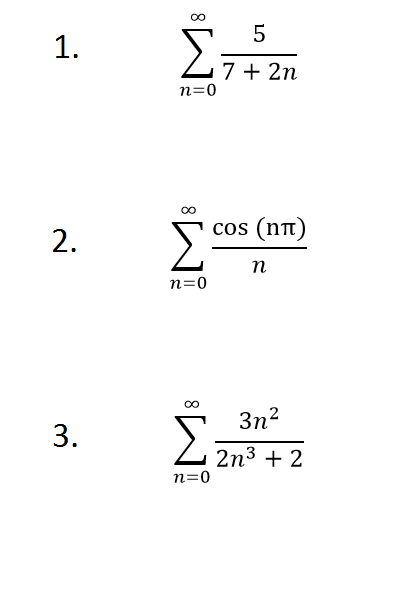## Solutions

1) The integral test can be used because the corresponding function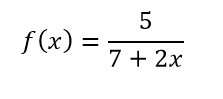is continuous, positive and decreasing. The only condition that may need to be checked is the decreasing part. We are only concerned about non-negative values of since our series begins at n=0. The function is decreasing because higher values of x result in a larger denominator.

Then, using the integral test, we have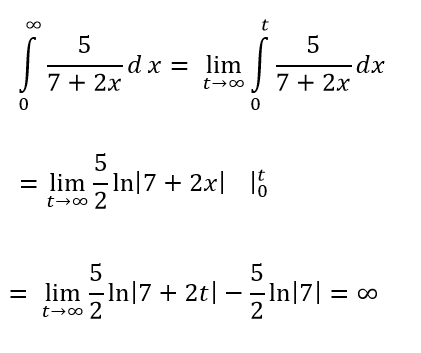The integral diverges and so the series also diverges.

2) The integral test cannot be used because the corresponding function (as well as the series) is not positive nor decreasing. The value of the numerator of the series alternates between 1 and -1, and so the integral test does not apply.

3) The integral test can be used because the corresponding function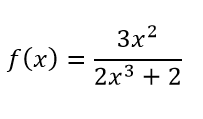is continuous, positive and decreasing. To check the decreasing condition, consider the derivative of the function: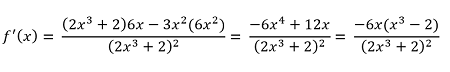which is negative when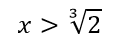and so the function is decreasing (at least eventually). Using the integral test, we have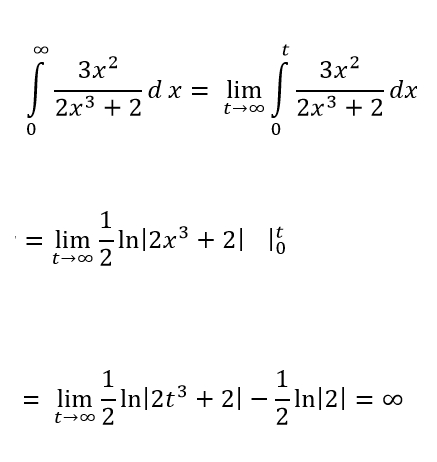The integral diverges and so the series also diverges.

#### How do you know if an integral converges?

To know if an integral converges, compute the antiderivative of the integrand, then take the limit of the result. If an integral converges, its limit will be finite and real-valued.

#### Can the integral test be used to test convergence?

Yes, in calculus, integral tests are used to test for convergence and divergence of integrals. These same integral tests can be used to test for the convergence or divergence of a series.

#### How do you test for integral convergence?

To test for integral convergence, first compute the antiderivative of the integrand. Next, take the limit of this antiderivative as the variable goes to infinity. If the result is finite and real-valued, the integral is convergent.

### Register to view this lesson

Are you a student or a teacher?